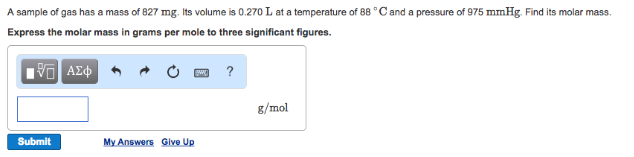# Problem: A sample of gas has a mass of 827 mg. Its volume is 0.270 L at a temperature of 88°C and a pressure of 975 mmHg. Find its molar mass. Express the molar mass in grams per mole to three significant figures.

###### FREE Expert Solution
94% (263 ratings)###### Problem Details

A sample of gas has a mass of 827 mg. Its volume is 0.270 L at a temperature of 88°C and a pressure of 975 mmHg. Find its molar mass. Express the molar mass in grams per mole to three significant figures.